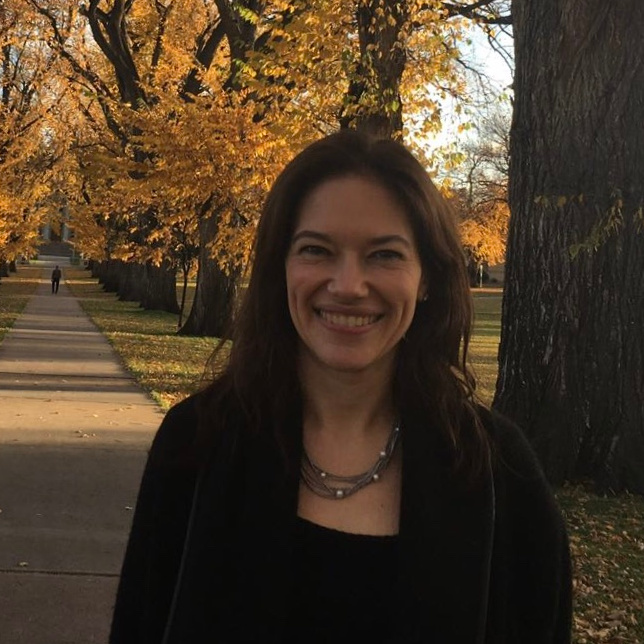# International School-conference in Algorithms, Combinatorics, and Complexity

24-28 May 2021
Zoom
Europe/Moscow timezone

## School in Algorithms, Combinatorics, and Complexity

### May 24 - May 28, 2021

An advanced school for young researchers featuring three minicourses in vibrant areas of mathematics and computer science.  The target audience includes graduate, master, and senior bachelor students of any mathematical speciality.

## Lecturers:Maria Chudnovsky Fedor Fomin Madhu Sudan Princeton University University of Bergen Harvard University

## Courses

### Forbidding Induced Subgraphs (Maria Chudnovsky)

The study of the structure of graphs with certain induced subgraphs forbidden has been an active area of research in graph theory in recent years, after the long standing Strong Perfect Graph Conjecture was proved using structural methods. The goal of this course is to cover some of the recent developments in the area. We will start with simple theorems about forbidden induced subgraphs, and work our way up to the latest research results. The topics will include perfect graphs, claw-free graphs, coloring graphs with forbidden induced subgraphs, algorithms for detecting induced subgraphs and others.

### Representative Families and Algorithms (Fedor Fomin)

The theorems of Bollobás (1965) and Lovász (1977) about set pairs are fundamental results in extremal set theory. In this tutorial, we discuss some algorithmic applications of these classical theorems. In particular, we learn about representative families and their role in kernelization,  enumeration, and parameterized algorithms.

### Highlights of Algorithmic Coding Theory (Madhu Sudan)

In this series of talks, we will quickly introduce the mathematical notions of an error-correcting code, and the associated notions of Encoding and Decoding Algorithms. After reviewing some of the classical results on the existence, construction and limits on error-correcting codes, we will zoom in on two results in the field.

1. The construction of capacity achieving codes (based on Folded Reed Solomon Codes) over large alphabets and their encoding and decoding: Given any error parameter $\delta \in [0, 1]$ and $\varepsilon > 0$, these codes achieve a rate $1 - \delta - \varepsilon$ and correct $\delta$ fraction of adversarial errors with alphabet size growing only with $\varepsilon$.
2. The construction of binary error correcting codes (Polar codes) that achieve Shannon capacity at small block lengths: I.e, given $\delta \in [0, 1 / 2]$ and $\varepsilon > 0$ correct delta fraction of random errors with rate $1 - \mathrm{h}(\delta) - \varepsilon$ at lengths $\mathrm{poly}(1 / \varepsilon)$. [Here $\mathrm{h}(p) = -p \log_2 p - (1 - p) \log_2 (1-p)$ is the binary entropy function].

Both results come with polynomial time algorithms!

## Videos

### Institutions participating in the organization of the event:

The school is supported by a grant from the Government of the Russian Federation, agreements 075-15-2019-1619 and 075-15-2019-1620.

Starts
Ends
Europe/Moscow
Zoom
 Representative Families and Algorithms-1.pdf Representative Families and Algorithms-2.pdf Representative Families and Algorithms-3.pdf
 A Few Words About the Proof Complexity.pdf Data Structures Meets Circuits and Cryptography.pdf Karchmer--Raz--Wigderson Conjecture.pdf Thursday talks.pdf